# 新能源汽车中预充电阻的选型及分析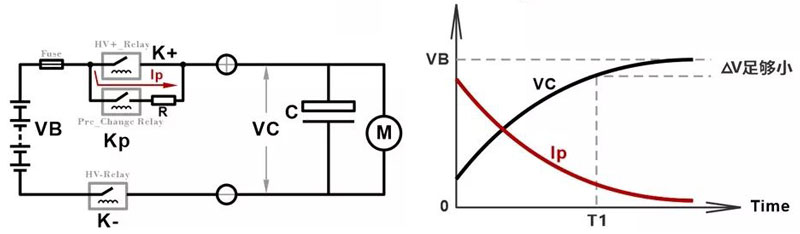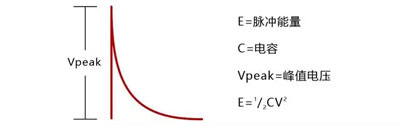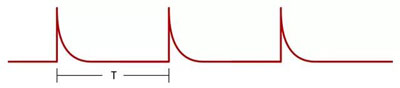T = RC * Ln[(Vbat - V0)/( Vbat - Vpre)]

R=预充电阻

C=负载端电容

Vbat=电池包电压

V0=负载端闭合高压前的电压（可表示为0）

Vpre=预充结束时负载端电压

T = RC * Ln10

E = 1/2CV2 = 442J

E= 442*5 = 2210J

R = T / (C * Ln10) = 300 / 4.60 = 65.2Ω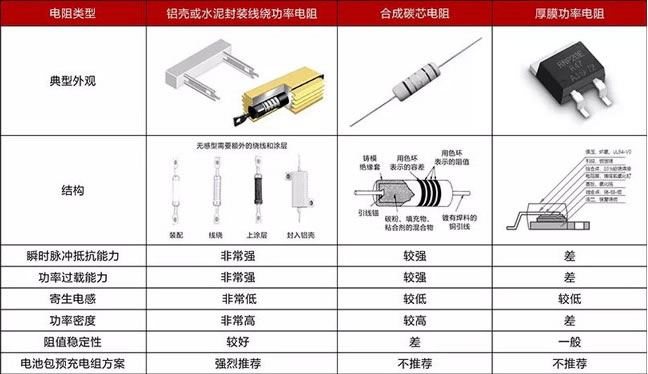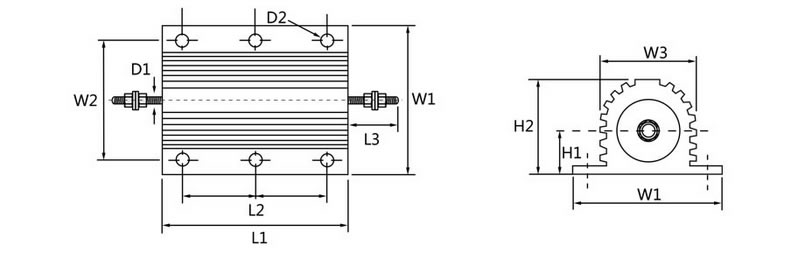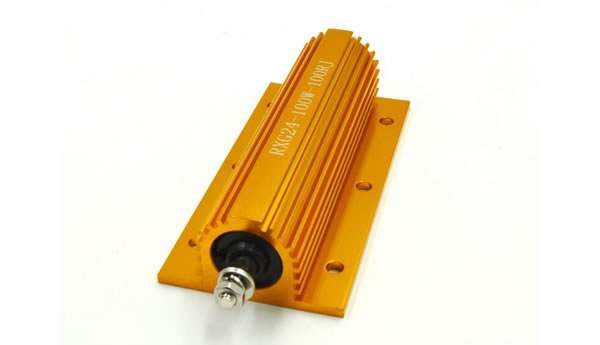### 客户留言

• 按需定制
量身定制最优方案和产品

• 1-4天货期
1-4天内超短货期

• 专业服务
专业工程市提供业务、技术服务

• 五年质保
五年质保等完善的售后服务